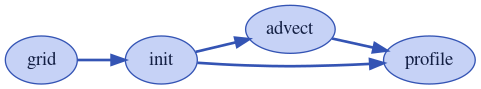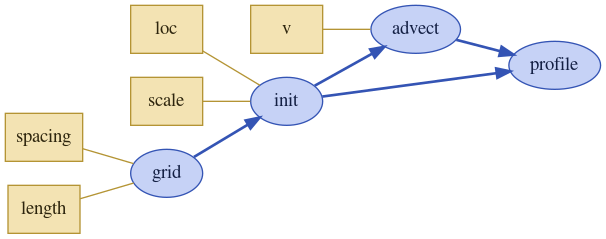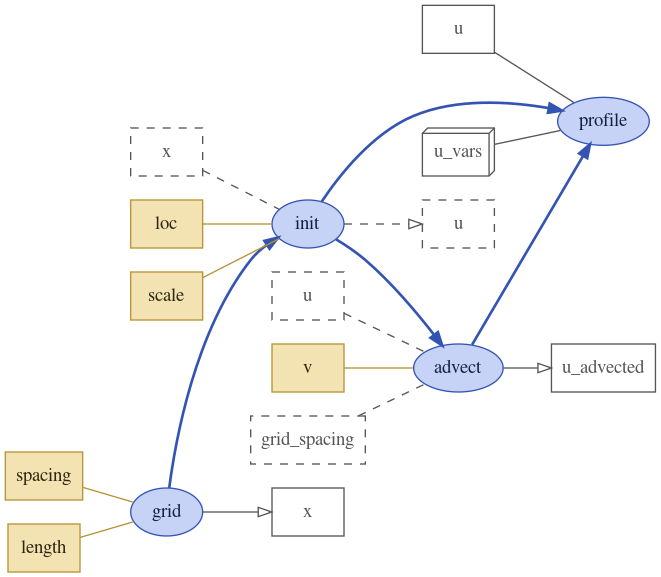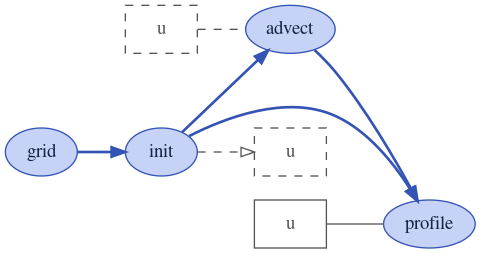# Inspect Models¶

Models may be complex and built from many processes and variables. To better explore those models, xarray-simlab provides many convenient ways to inspect and auto-document `Model` objects.

As an example we’ll use here the object `advect_model` which has been created in the previous Section Create and Modify Models of this user guide.

```In : import xsimlab as xs
```

## Inspect model inputs¶

Model repr already gives information about the number and names of processes and their variables that need an input value (if any):

```In : advect_model
Out:
<xsimlab.Model (4 processes, 5 inputs)>
grid
spacing     [in] uniform spacing
length      [in] total length
init
loc         [in] location of initial pulse
scale       [in] scale of initial pulse
v           [in] () or ('x',) velocity
profile
```

For each input, a one-line summary is shown with the intent (either ‘in’ or ‘inout’) as well as the dimension labels for inputs that don’t expect a scalar value only. If provided, a short description is also displayed in the summary.

The convenient property `input_vars` of Model returns all inputs as a list of 2-length tuples with process and variable names, respectively.

```In : advect_model.input_vars
Out:
[('grid', 'spacing'),
('grid', 'length'),
('init', 'loc'),
('init', 'scale'),
```

`input_vars_dict` returns all inputs grouped by process, as a dictionary:

```In : advect_model.input_vars_dict
Out: {'grid': ['spacing', 'length'], 'init': ['loc', 'scale'], 'advect': ['v']}
```

## Inspect processes and variables¶

For deeper inspection, Model objects support both dict-like and attribute-like access to their processes, e.g.,

```In : advect_model['advect']
Out:
Variables:
v                [in] () or ('x',) velocity
grid_spacing     [in] <--- grid.spacing
u                [in] <--- profile.u
Simulation stages:
run_step

Out:
<UniformGrid1D 'grid' (xsimlab process)>
Variables:
spacing     [in] uniform spacing
length      [in] total length
x          [out] ('x',)
Simulation stages:
initialize```

As shown here above, process repr includes:

• the name to the process class and the name of the process in the model (top line) ;

• a “Variables” section with all variables declared in the process (not only model inputs) including one-line summaries that depend on their type (i.e., `variable`, `foreign`, `group`, etc.) ;

• a “Simulation stages” section with the stages that are implemented in the process.

It is also possible to inspect a process class taken individually with `process_info()`:

```In : xs.process_info(ProfileU)
<ProfileU  (xsimlab process)>
Variables:
u_vars      [in] <--- group 'u_vars'
u        [inout] ('x',) quantity u
Simulation stages:
run_step
finalize_step
```

Similarly, `variable_info()` allows inspection at the variable level:

```In : xs.variable_info(ProfileU, 'u')
Quantity u

Variable properties:

- type : ``variable``
- intent : ``inout``
- dimensions : ('x',)

Other attributes:

- units : m

Iterable of all variables that belong to group 'u_vars'

Variable properties:

- type : ``group``
- intent : ``in``
- default value : ()```

Alternatively, you can look at the auto-generated docstrings of a process class (configurable via the `autodoc` parameter of `process()`):

```In : ProfileU?
Init signature: ProfileU(*, u_vars=(), u) -> None
Docstring:
Compute the evolution of the profile of quantity `u`.

Attributes
----------
u_vars : :class:`attr.Attribute`
Iterable of all variables that belong to group 'u_vars'

Variable properties:

- type : ``group``
- intent : ``in``
- default value : ()

u : :class:`attr.Attribute`
Quantity u

Variable properties:

- type : ``variable``
- intent : ``inout``
- dimensions : ('x',)

Other attributes:

- units : m
Init docstring: Method generated by attrs for class ProfileU.
Type:           type
Subclasses:     ProfileU
```

As well as the auto-generated docstrings for each variable (only accessible from Model objects), e.g.,

```In : advect_model.profile.u?
Type:        property
String form: <property object at 0x7fa9b8f6aa10>
Docstring:
Quantity u

Variable properties:

- type : ``variable``
- intent : ``inout``
- dimensions : ('x',)

Other attributes:

- units : m
```

Like `input_vars` and `input_vars_dict`, Model properties `all_vars` and `all_vars_dict` are available for all model variables, not only inputs.

## Visualize models as graphs¶

It is possible to visualize a model and its processes as a directed graph (note: this requires installing Graphviz and its Python bindings, which both can be found on conda-forge):

```In : advect_model.visualize();
````show_inputs` option allows to show model input variables as yellow square nodes linked to their corresponding processes:

```In : advect_model.visualize(show_inputs=True);
````show_variables` option allows to show the other variables as white square nodes:

```In : advect_model.visualize(show_inputs=True, show_variables=True);
```Nodes with solid border correspond to regular variables while nodes with dashed border correspond to foreign variables. 3d-box nodes correspond group variables. Variables connected to their process with an arrow have a value computed by the process itself (i.e., `intent='out'`).

A third option `show_only_variable` allows to show only one given variable and all its references in other processes, e.g.,

```In : advect_model.visualize(show_only_variable=('profile', 'u'));
```Note that there is another function `dot_graph` available in module `xsimlab.dot` which produces similar graphs and which has a few more options.